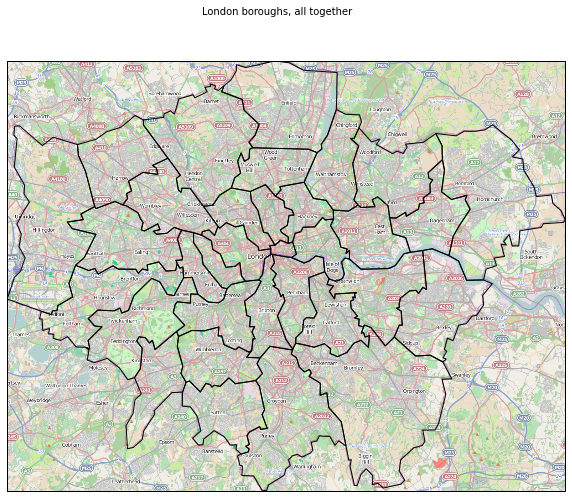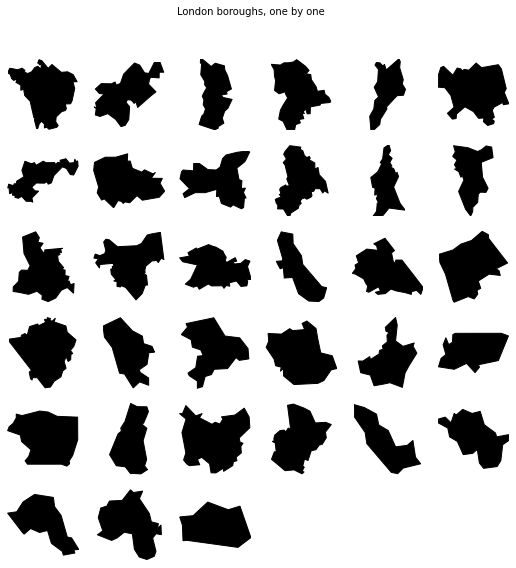Fun with PySAL and cartopy: London boroughs, one by one¶

This short example shows how one can easily use cartopy to beautifully plot polygons from PySAL. Read your data with PySAL, convert them to matplotlib with its viz module and set the right projection and other geo goodies with cartopy.

This notebook (and the data required to run it), are hosted as a gist on this link.

In :
%matplotlib inline
import matplotlib.pyplot as plt
import pysal as ps
import numpy as np
from pysal.contrib.viz import mapping as maps
import cartopy.io.img_tiles as cimgt
import cartopy.crs as ccrs


Data¶

London borough data from Robin Lovelace's tutorial on R (link). The shapefile LondonBoroughs is reprojected to lon/lat and renamed boroughs. For convenience, the shapefile is attached to the gist where this notebook is hosted.

Overall map¶

In :
shp = ps.open('boroughs.shp')
bg = cimgt.OSM()

In :
extent = [shp.bbox, shp.bbox, shp.bbox, shp.bbox]
boros = maps.map_poly_shp(shp)
boros.set_transform(ccrs.Geodetic())
boros.set_facecolor('none')

f = plt.figure(figsize=(10, 8))

ax = plt.axes(projection=bg.crs)
ax.set_extent(extent)

f.suptitle('London boroughs, all together')

plt.show()Boroughs¶

In :
f = plt.figure(figsize=(9, 9))

for i, boro in enumerate(shp):
extent = [boro.bbox, boro.bbox, boro.bbox, boro.bbox]
boroP = maps.map_poly_shp([boro], setup=False)
boroP.set_transform(ccrs.PlateCarree())
boroP.set_facecolor('k')

ax = plt.subplot(6, 6, i+1, projection=ccrs.OSGB())
ax.set_extent(extent)

ax.outline_patch.set_visible(False)

f.suptitle('London boroughs, one by one')
plt.show()Test

In :
from IPython.core.display import HTML
def css_styling():